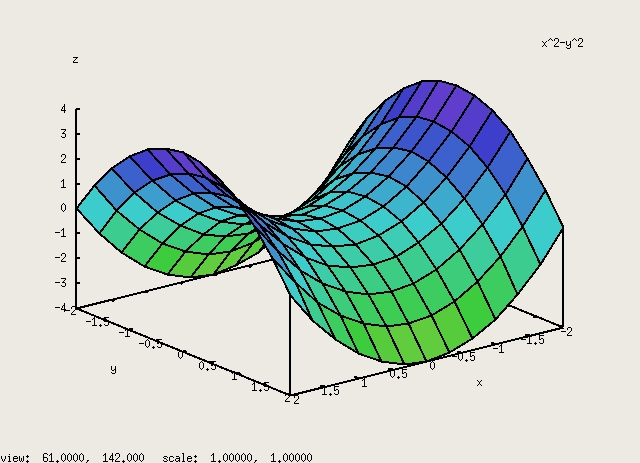# DEPARTMENT OF MATHEMATICS

## Maxima

Maxima is a system for the manipulation of symbolic and numerical expressions, including differentiation, integration, Taylor series, Laplace transforms, ordinary differential equations, systems of linear equations, polynomials, and sets, lists, vectors, matrices, and tensors. Maxima yields high precision numeric results by using exact fractions, arbitrary precision integers, and variable precision floating point numbers. Maxima can plot functions and data in two and three dimensions.

Similar in some regards to Maple and Mathematica but free.